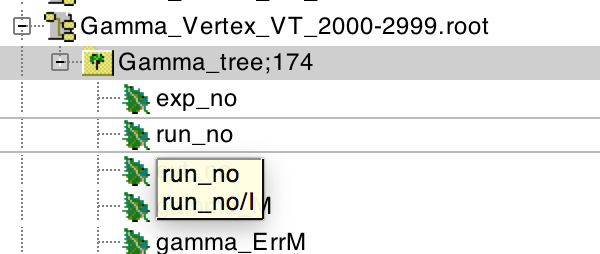# Scaling 1D and 2D Histograms using Trees and Tuples

ROOT Version: root_v6.10.02
Platform: Mac OSX Terminal
Compiler: Not Provided

Hi,

I’m trying to Scale/Normalize basic 1D and 2D histograms I’ve created using TNtuples.
My basic MacroCode before styling and such to Draw these two types of histograms with Cuts would be:

void ScaleExample(){

``````TFile* f1 = TFile::Open("FilePath/FileName.root");

TNtuple* T_Gamma_Tree =  (TNtuple*) f1->Get("Gamma_tree");

T_Gamma_Tree -> Draw("gamma_M","gamma_M>0&&gamma_M<20","");
//T_Gamma_Tree -> Draw("gamma_X:gamma_Z","(gamma_Z>-70&&gamma_Z<70)&&gamma_Rho<15","");
``````

}

These are created elsewhere and I receive them as the image below, so I need to scale them from this stage.

I know for normal Histograms using TH1F, you could use a line kind of like this:

TH1F *h1 = new TH1F(“h1”, “h1”, 100, -5, 5);
h1->FillRandom(“gaus”);
Double_t norm = h1->GetEntries();
h1->Scale(1/norm);

But I don’t Know how to apply that to my TNtuple (TTree) style of writing.

The attached file is what the NTuple looks like.
The gamma_M Histogram is just your normal binned 1D histogram.

Thanks!After:

``````T_Gamma_Tree -> Draw("gamma_M","gamma_M>0&&gamma_M<20","");
``````

The histogram generated is called `htemp`. You can do the scaling on it.

``````htemp->Scale(1/norm);
``````

This topic was automatically closed 14 days after the last reply. New replies are no longer allowed.